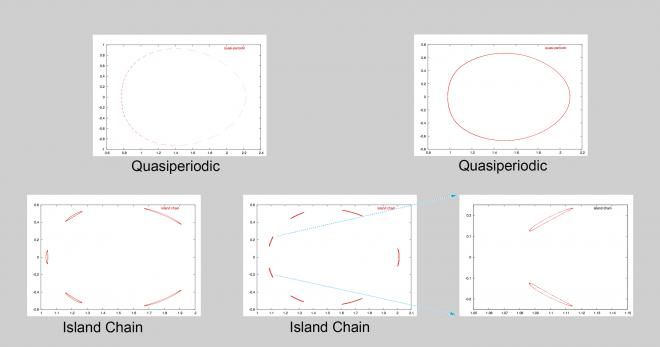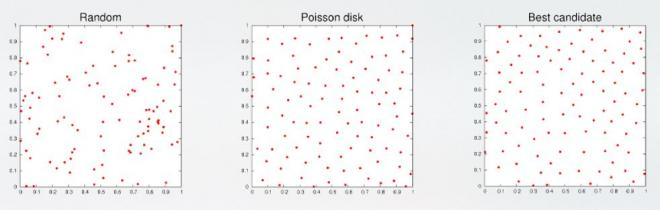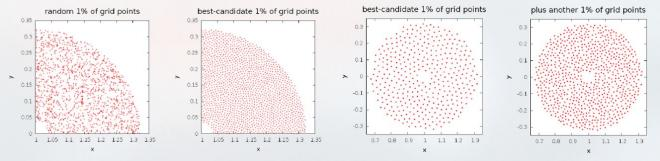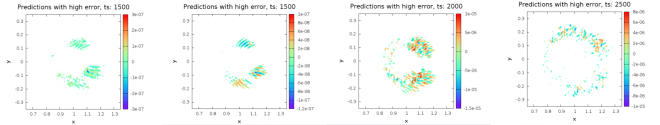The increasing size of scientific datasets presents challenges for fully exploring the data and evaluating an analysis approach. If the spatio-temporal variation in scientific data is not considered in the analysis, incorrect or incomplete conclusions could be drawn. In the IDEALS project, statistical and machine learning techniques are combined to give scientists and data analysts the same level of confidence in the analysis of large-scale data as they have in the analysis of small datasets, resulting in improved scientific and policy decisions.

For example, if subtle differences among orbits in a Poincaré plot (Figure 1) were overlooked when planning an analysis approach to automatically assign an orbit type based on the shape traced out by a sequence of points, incorrect conclusions would have been drawn from the data. This problem worsens as the size of the data increases.Figure 1. A small data set illustrating the subtle variation in orbits. A quasi-periodic orbit can appear as a closed curve (top left) or a curve with gaps that are filled as more points are added (top right). An island-chain orbit also has gaps, but each segment has a width (bottom left) and the gaps never get filled as more points are added. However, an island-chain orbit with very thin segments (bottom middle) can appear as a quasi-periodic orbit with gaps. This subtle difference is only visible when we zoom-in to the segments (bottom right). (Click to enlarge.)

## Intelligent Sub-Sampling

A simple and practical way to explore a large dataset is to start with a small sample. For 100 samples in a two-dimensional domain, random sampling over- or under-samples the region. Poisson disk sampling spreads the samples more evenly, but new samples are difficult to add. The best-candidate method gives good coverage and can be modified to meet our needs.Figure 2. The best-candidate sampling method provides a good spatial distribution of points and modification advantages over random and Poisson disk sampling methods. (Click to enlarge.)

## One-Pass Sampling Algorithm

We modified the best-candidate algorithm to select a smaller subset of points, from an existing set, using a single pass through the data. If the initial set of samples is insufficient for analysis, the user can incrementally add new samples while maintaining the spread among selected samples.Figure 3. Far left: Random sampling of 1% of the grid points in a large data set of 591,745 grid points. Second from left: Best-candidate method. Only a quarter of the data set is shown. Third from left: 1% of the grid points of a smaller data set with 39,693 grid points. Far right: With an additional 1% of samples. (Click to enlarge.)

## Finding Interesting Regions in the Data

Having selected a subset of the grid points, we can use machine learning and statistical techniques to find “interesting” regions in the data. The single-pass variants of these algorithms can quickly indicate which regions of a large data set should be explored further. For example, we can use locally-weighted kernel regression and the values of the variable of interest at the 2% subset samples to predict the values at the remaining 98% of the data. A high error in prediction occurs where there is a lot of variation in the data, indicating an interesting region.Figure 4. The first two columns correspond to high-error points in the predicted output variable at time step 1500, generated using a random subset and the best candidate subset. The third and fourth column are high-error points for time steps 2000 and 2500 using the best candidate subset. The original values of the output at the three times steps are shown in the leaderboard image above. (Click to enlarge.)